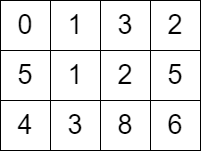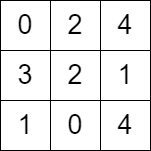## 题目描述

leetcode 6366. 在网格图中访问一个格子的最少时间```输入：grid = [[0,1,3,2],[5,1,2,5],[4,3,8,6]]

- 时刻 t = 0 ，我们在格子 (0,0) 。
- 时刻 t = 1 ，我们移动到格子 (0,1) ，可以移动的原因是 grid <= 1 。
- 时刻 t = 2 ，我们移动到格子 (1,1) ，可以移动的原因是 grid <= 2 。
- 时刻 t = 3 ，我们移动到格子 (1,2) ，可以移动的原因是 grid <= 3 。
- 时刻 t = 4 ，我们移动到格子 (1,1) ，可以移动的原因是 grid <= 4 。
- 时刻 t = 5 ，我们移动到格子 (1,2) ，可以移动的原因是 grid <= 5 。
- 时刻 t = 6 ，我们移动到格子 (1,3) ，可以移动的原因是 grid <= 6 。
- 时刻 t = 7 ，我们移动到格子 (2,3) ，可以移动的原因是 grid <= 7 。

``````输入：grid = [[0,2,4],[3,2,1],[1,0,4]]

```

• `m == grid.length`
• `n == grid[i].length`
• `2 <= m, n <= 1000`
• `4 <= m * n <= 105`
• `0 <= grid[i][j] <= 105`
• `grid == 0`